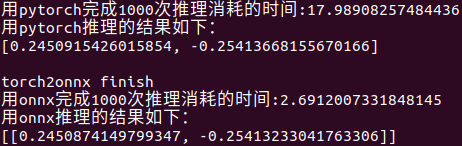1. 环境准备

2. 测试过程

3. 测试结果与分析

## 1. 环境准备

对比时间，和模型训练的环境相同，可能额外要安装的包是onnxruntime.

``````pip install onnxruntime      # for cpu
pip install onnxruntime-gpu  # for gpu``````

## 2. 测试过程

直接上代码吧，代码就是最好的解释。

``````import cv2
import time
import torch
import numpy as np
from torch.nn import DataParallel
from MobileNetV2 import mobilenet_v2
from collections import OrderedDict
from torchvision import transforms as T
import onnxruntime as rt

def torch2onnx(model, save_path):
model.eval()
data = torch.rand(1,3,256,256)
input_names = ['input']
output_names = ['out']
torch.onnx.export(model,
data,
save_path,
export_params=True,
opset_version=11,
input_names=input_names,
output_names=output_names)
print("torch2onnx finish")

def img_process(img_path):
normalize = T.Normalize(mean = [0.5, 0.5, 0.5],
std = [0.5, 0.5, 0.5])
transforms = T.Compose([T.ToTensor(),
normalize])
img = cv2.cvtColor(img, cv2.COLOR_BGR2RGB)
img = cv2.resize(img, (256, 256))
img = transforms(img)
img = img.unsqueeze(0)
return img

def onnx_runtime(img):
sess = rt.InferenceSession("mobilenet-v2.onnx")
input_name = sess.get_inputs().name
output_name = sess.get_outputs().name
t0 = time.time()
for i in range(1000):
pred_onnx = sess.run([output_name], {input_name:np.array(img)})
t1 = time.time()
print("用onnx完成1000次推理消耗的时间:%s" % (t1-t0))
print("用onnx推理的结果如下：")
print(pred_onnx.tolist())

new_state_dict = OrderedDict()
for k, v in state_dict.items():
name = k
if name.startswith("module."):
name = name[7:]
new_state_dict[name] = v
model.eval()
return model

if __name__ == "__main__":
device = torch.device("cuda" if torch.cuda.is_available() else "cpu")
model = mobilenet_v2().to(device)
model_pth = "./mobilenet-v2.pth"

img = img_process("test.jpg")
t0 = time.time()
for i in range(1000):
outputs = model(img)
t1 = time.time()
print("用pytorch完成1000次推理消耗的时间:%s" % (t1-t0))
print("用pytorch推理的结果如下：")
print(outputs.detach().tolist())
print()

torch2onnx(model, "mobilenet-v2.onnx")

onnx_runtime(img)
``````

## 3. 测试结果与分析

运行上面代码，输出如下(我是用cpu跑的结果)：可以看到用pytorch和onnx的推理结果几乎相同，完全可以接受。然而，用onnx的推理速度是pytorch的好几倍。

OK，就是这么简单~### if-else嵌套太深？教你一个新手都能掌握的设计模式搞定！-程序员宅基地

△Hollis, 一个对Coding有着独特追求的人△这是Hollis的第259篇原创分享作者 l 南山狮来源 l Hollis（ID：hollischuang）我也不用设计模式很多...

### 目标检测深度学习方法综述（一）_深度学习的目标检测最新方法-程序员宅基地

0.前言从去年九月份以来，我断断续续的接触并了解了深度学习中目标检测方面的知识。读了几篇论文，也尝试着跑了几个代码，对目标检测领域的深度学习方法有了大致的了解，一直准备写一篇综述性的学习报告，来总结我所学到，看到的知识点。但由于所学太过于零碎，不成体系，一直没有动手整理。直到前两天我导让我将18年之后比较典型的神经网络模型总结一哈，我这下定决心，准备将我这大半年所学到的关于深度学习目标检测的知识..._深度学习的目标检测最新方法

### python绘制等值线图_python,matplotlib_python菜鸟求助，使用matplotlib 绘制contour等高线图，z为2D数组？，python,matplotlib - php...-程序员宅基地

python菜鸟求助，使用matplotlib 绘制contour等高线图，z为2D数组？有一系列点坐标如下所示：x,y,z74,781,51373,731,111321,1791,280,1787,41049,2127,121647,2728,62883,3617,152383,3692,72708,2295,222933,1767,74233,895,64043,1895,14想通过conto..._contourf的input z must be2d

### CountVectorizer参数-程序员宅基地

https://zhuanlan.zhihu.com/p/37644086